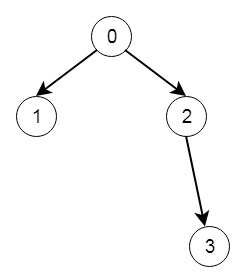# Validate Binary Tree Nodes in C++

Suppose we have n binary tree nodes numbered from 0 to n - 1 where node I has two children leftChild[i] and rightChild[i], we have to say true if and only if all the given nodes form exactly one valid binary tree. When node i has no left child then leftChild[i] will equal -1, similarly for the right child. We have to keep in mind that the nodes have no values and that we only use the node numbers in this problem. So if the input is like −Then the output will be true.

To solve this, we will follow these steps −

• Define a method called dfs, this will take leftChild, rightChild and visited

• if node n is visited, then return false

• insert node n into visited set

• set ret := true

• if leftChild of n is not -1, then ret := ret AND dfs(leftChild[node], leftChild, rightChild, visited)

• if rightChild of n is not -1, then ret := ret AND dfs(rightChild[node], leftChild, rightChild, visited)

• return ret

• The main method will be like −

• ret := dfs(0, leftChild, rightChild, visited)

• set allVisited := false

• for I in range 0 to n – 1

• if i is not visited, then return false

• return ret

## Example (C++)

Let us see the following implementation to get a better understanding −

Live Demo

#include <bits/stdc++.h>
using namespace std;
class Solution {
public:
bool dfs(int node, vector <int>& leftChild, vector <int>& rightChild, set <int>& visited){
if(visited.count(node)) return false;
visited.insert(node);
bool ret = true;
if(leftChild[node] != -1){
ret &= dfs(leftChild[node], leftChild, rightChild, visited);
}
if(rightChild[node] != -1){
ret &= dfs(rightChild[node], leftChild, rightChild, visited);
}
return ret;
}
bool validateBinaryTreeNodes(int n, vector<int>& leftChild, vector<int>& rightChild) {
set <int> visited;
bool ret = dfs(0, leftChild, rightChild, visited);
bool allVisited = true;
for(int i = 0; i < n; i++){
if(!visited.count(i))return false;
}
return ret;
}
};
main(){
vector<int> v1 = {1,-1,3,-1}, v2 = {2,-1,-1,-1};
Solution ob;
cout << (ob.validateBinaryTreeNodes(4, v1, v2));
}

## Input

4
[1,-1,3,-1]
[2,-1,-1,-1]

## Output

1

Updated on: 29-Apr-2020

174 Views